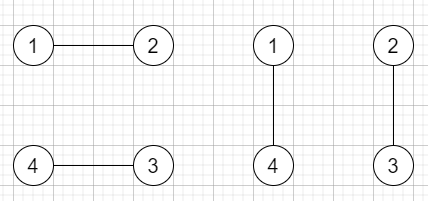# Handshakes That Don't Cross in C++

Suppose we have an even number of people n that stand around a circle and each person shakes hands with someone else, so that there will be n / 2 handshakes total. We have to find the number of ways these handshakes could occur such that none of the handshakes cross. The answers may be very large so return the answer mod 10^9 + 7.

So, if the input is like n = 2, then the output will be 1To solve this, we will follow these steps −

• m := 10^9 + 7

• Define an array dp of size (n+1)

• dp := 1

• for initialize i := 0, when i <= n, update i := i + 2, do −

• for initialize j := 0, when j <= i-2, update j := j + 2, do −

• dp[i] := dp[i] + (dp[j] mod m * dp[i - 2 - j] mod m)

• dp[i] := dp[i] mod m

• return dp[n] mod m

Let us see the following implementation to get better understanding −

## Example

Live Demo

#include <bits/stdc++.h>
using namespace std;
const int m = 1e9+7;
typedef long long int lli;
class Solution {
public:
int numberOfWays(int n) {
vector <lli> dp(n+1);
dp = 1;
for(int i = 0; i <= n; i+=2 ){
for(int j =0 ; j <= i-2; j+=2){
dp[i] += (dp[j]%m * dp[i-2-j]%m)%m;
dp[i]%=m;
}
}
return dp[n]%m;
}
};
main(){
Solution ob;
cout << (ob.numberOfWays(2));
}

## Input

2

## Output

1